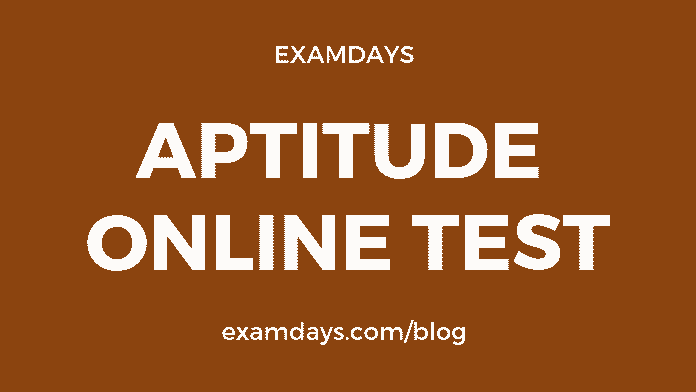# Aptitude Online Test Quiz # 5 with Answers

0# Aptitude Online Test

General quantitative aptitude online test is more important for the various examinations, such as SSC, Railways RRB, PSU, State PSC, IBPS Banks, and other Government notifications, The aptitude questions practice make sense in the examination purpose, candidates should practice as many of questions and different models of questions.

Q1. Three pipes A, B and C can fill a tank in 6 hours. After working at it together for 2 hours, C is closed and A and B can fill the remaining part in 7 hours. The number of hours taken by C alone to fill the tank is:

1. 10
2. 12
3. 14
4. 16

14

Q2. Mahesh covers a distance by cycle at 18 km/h. He returns to the starting point in a bus at a speed of 45 km/h. Find the average speed for the entire journey.

1. 27.39 km/hr
2. 25.71 km/hr
3. 27 km/hr
4. 29 km/hr

25.71 km/hr

Q3. Price of each article of type P, Q, and R is Rs. 300, Rs. 180 and Rs. 120 respectively. Suresh buys articles of each type in the ratio 3:2:3 in Rs. 6480. How many articles of type Q did he purchase?

1. 2
2. 4
3. 6
4. 8
5. 10

8

Q4. How many 8’s are present in the following sequence of numbers which are exactly divisible by both its preceding and following numbers?

3 8 6 8 8 7 6 8 3 4 8 2 5 6 2 8 2 4 8 6 3 7 4 8 4 5 8 4

1. 2
2. 4
3. 6
4. 8

4

Q5. Two houses are in front of each other. Both have chimneys on their top. The line joining the chimneys makes an angle of 45° with the ground. How far are the houses from each other if one house is 25m and other is 10m in height?

1. 18m
2. 16m
3. 15m
4. 19m

15m

Q6. Two pipes A and B can fill a tank in 15 minutes and 20 minutes respectively. Both the pipes are opened together but after 4 minutes, pipe A is turned off. What is the total time required to fill the tank?

1. 12 min. 20 sec.
2. 12 min. 40 sec.
3. 14 min. 20 sec.
4. 14 min. 40 sec.

14 min. 40 sec.

Q7.  In a division sum, the remainder is 6 and the divisor is 5 times the quotient and is obtained by adding 2 to the thrice of the remainder. The dividend is:

1. 89
2. 86
3. 88
4. 85

86

Q8. X persons stand on the circumference of a circle at distinct points. Each possible pair of persons, not standing next to each other, sings a two-minute song one pair after the other. If the total time taken for singing is 28 minutes, what is X?

1. 6
2. 5
3. 7
4. 9
5. 11

7

Q9. When you reverse the digits of the number 13, the number increases by 18. How many other two-digit numbers increase by 18 when their digits are reversed?

1. 2
2. 4
3. 6
4. 8
5. 10

6

Q10. What is the sum of this series 1 + 1 + 2 + 8 + 3+ 27 + 4 + 64 +_______10 + 1000?

1. 308
2. 3008
3. 3800
4. 3080